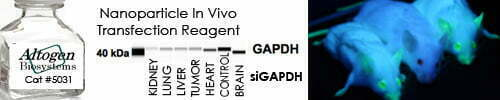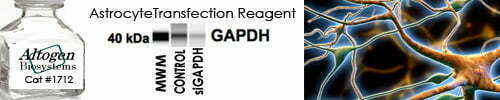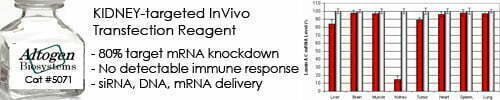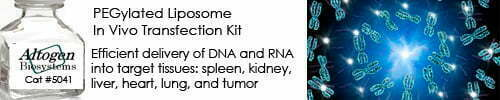••••Shop Products

# Units of Measurement Conversions

Reference tables for common units of measurement and their equivalents and conversion factors.

 Weight 1 g =103 mg = 10-3 kg = 106 µg = 3.53 x 10-2 oz 1 oz = 28.3 g 1 lb = 16 oz = 0.45 kg 1 kg = 103 g = 2.2 lb
 Fluids 15 ggts = 1 mL = 10-3 L 1 cup = 8 oz = 236.5 mL 1 pt = 16 oz = 473 mL = 2 cups 1 qt = 32 oz = 0.946 L = 4 cups = 2 pts 1 gal = 4 qts = 3.785 L 1 glass = 8 oz 1 teacup = 6 oz =1 gtt = 1 minim = 0.06 mL 1 fldr = 3.96 mL = 60 minims 1 floz = 8 fldr = 6 tsp = 2 tbsp = 29.57 mL 1 dr = 27 11/32 gr 1 oz = 16 dr = 2 tbsp = 6 tsp 1 tsp = 60 gtt = 1 1/3 fldr = 5 mL 1 tbsp = 3 tsp = 4 fldr = ½ oz = 15 mL 1 mL = 0.27 fldr = 16.23 minims
 Length 1 mm = 0.039 in = 0.1 cm = 107 Å 1 in = 2.54 cm 1 yard = 3 ft = 36 in 1 m = 103 mm = 100 cm = 1.09 yards = 3.28 ft 1 dm = 3.94 in 1 dam = 32.81 ft 1 km = 0.62 mi = 3280.1 ft
 Temperature °C = 5/9(°F – 32) °F = (°C x 1.8) + 32 K = °C + 273 0°C = 32°F (freezing water) 35°C = 95°F 100°C = 212°F (boiling water)
 Energy 1 J = 107 erg = 0.239 cal 1 cal = 4.184 J
 Pressure 1 torr = 1 mm Hg = 1.32 x 10-3 atm = 1.333 x 102 Pa 1 atm = 758 torr = 1.01 x 105 Pa
 Radioactivity 1 Ci = 3.7 x 1010 dps = 37 GBq 1,000 dpm = 16.7 Bq

Sorry, comments are closed for this post.